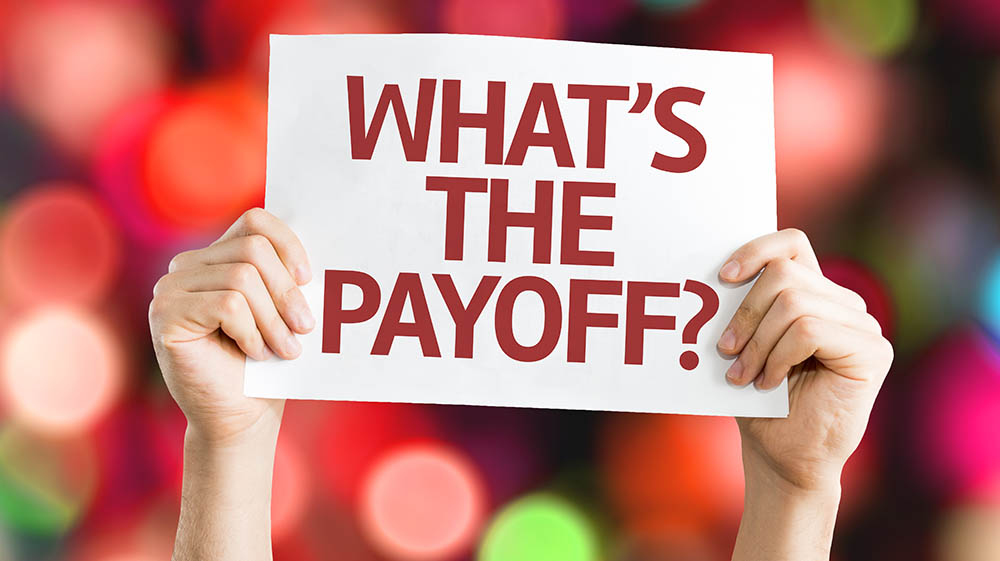The other day I was thinking about how we use Cash on Cash Return (CCR) to filter and compare potential rental property investments, and how that metric is only useful to understand the first year performance of a potential long-term buy-and-hold deal.

This post explains the limitation of Cash on Cash Return (CCR) beyond the first year, and why you should use Internal Rate of Return (IRR) to analyze the long-term potential of your rental property investments instead.

## Starting at the Beginning: What is ROI?

ROI is the rate of return of your total investment.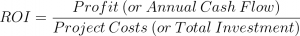Generally, ROI is used for short-term investments such as flips where you can calculate all of the profit from any incomes including the final sale event, all of which usually occur in the span of less than a year. This makes profit easy to calculate.

When working with long-term investments, however, you have to establish a time period for your ROI calculation, which is usually a year, and use the cash flows from that year to calculate the profit side of the equation.

## What is Cash-on-Cash Return?

Cash-on-cash return (CCR) is a ratio of before-tax cash flow to the amount of out of pocket cash invested.This is almost the same as return on investment (ROI) with the exception that ROI measures the return on the entire amount invested, whereas CCR is useful to identify your leveraged returns, by only calculating the returns on equity that you put into the deal. This is why its other name is the Equity Dividend Rate.

CCR is used to show how your leveraged returns change based only on your invested equity in the project, as opposed to the total costs of the project.

An interesting result of this is that if you were to not use leverage, then CCR and ROI are the same. This is because you have 100% equity in the project, meaning that the Cash Invested from the denominator of the CCR formula is the same as Total Investment of project costs as in the ROI formula.

## Example Scenario of Unleveraged and Leveraged ROI and CCR

Let’s take an example scenario where you have the opportunity to use leverage to purchase, rehab, and hold a \$100,000 property investment with the following numbers:

 Investment Monthly Cash Flow Purchase Price \$75,000 Gross Income \$1,200 Purchase Costs \$3,000 Vacancy (10%) (\$120) Rehab Costs \$22,000 Property Mgmt. (10%) (\$120) Total Costs \$100,000 Utilities (\$200) Reserves (10%) (\$120) Financing Unleveraged Cash Flow \$640 70% LTV \$70,000 Monthly Debt Service (30 Year FHA @ 4.5%) (\$355) Equity \$30,000 Leveraged Cash Flow \$285

What are the Unleveraged and Leveraged ROI and CCR of this Property?

#### Unleveraged

As expected because your total equity equals your total costs, the ROI and CCR yield the same result.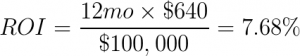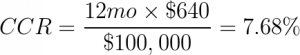#### Leveraged

Using the leveraged cash flow numbers you can see that ROI goes down, and CCR goes up.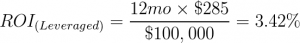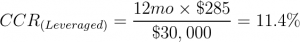So which metric do you choose? Is it better to lever or not?

A leveraged ROI calculation is not a very useful metric. What you would be doing in that instance is calculating leveraged cash flow on an unleveraged total cost, which is misleading because you didn’t actually invest the entire cost of the project.

Instead, it is better to use CCR to compare leveraged deals, and to help you determine how much leverage to use to get just the right return on your invested equity.

But only for the first year.

Why?

For long-term investments Cash On Cash Return, (and ROI) should only be calculated for the first year because of something called the time value of money.

## Time Value of Money

The time value of money is a concept, which states that money available today is more valuable than money received in the future. This happens because over time money can earn interest or can be invested, and so money invested later, doesn’t have as much time to work.  Additionally, money becomes less valuable due to inflation.

In the case of performing a Cash on Cash Return for future years, if you were to use the cash flows from several years into the future, without discounting those cash flows to present value, you would be overvaluing the investment.

What you need to run instead is a cash flow analysis on your investment that takes the time value of money into account.

For this, you should understand Future Value, Present Value, Net Present Value, and finally analyze the investment using the Internal Rate of Return (IRR) to get a much more accurate depiction of the potential performance of an investment.

### What is Future Value?

Money invested today will increase in value over time at a particular rate of return that’s true for that investment. The formula to determine the Future Value of money is as follows: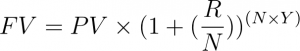Where:

FV: Future Value of Investment

PV: Present Value of Cash Flow

R: The interest rate at which the investment is expected to grow each year

N: The number of compounding periods per year

Y: The number of years that the investment is held

So for an example, let’s say that you made an investment in stocks of \$100,000 over 7 years, at a growth rate of 10%, compounded monthly. You would end up with the following future values for that \$100,000 in each year:

If you bought \$100,000 in stocks with a growth rate of 10% each year, then at year 7 your investment would be worth twice as much in today’s dollars at \$200,792.

You can use this same formula to solve for the Present Value of money based on that same interest rate, also called the discount rate.

### What is Present Value?

The Present Value of money is the value of an expected future income stream in today’s dollars based on a discount rate. The discount rate is a rate of return that you would expect to earn on that money if you invested it.

Let’s assume that you expect your cash flow from rents to be \$10,000 each year for the next 7 years. In order to understand the discounting of your future cash flows, you need to find out how much \$10,000 that was earned in a particular year, is worth in today’s dollars.

In order to do this, you can solve for Present Value by rearranging the same formula that you used to determine Future Value.

The formula for Present Value is thus: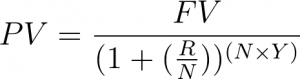Using the example scenario, the expected future cash flows of \$10,000 each year discounted at a rate of 10%, compounded monthly, the value of each year of those future cash flows in today’s dollars looks like this:

As you can see, if you expected a rental cash flow event of \$10,000 in year 6, then that money would be worth only \$5,502 in today’s dollars.

This means that if you do not grow your future expected cash flows, then their value will be diminished over time making other investments potentially more worthwhile.

## What is Net Present Value (NPV)?

Net Present Value is the difference between the Present Value of cash inflows, and the Present Value of cash outflows discounting each cash flow to the present using a pre-defined discount rate, over a particular period.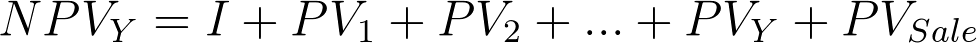A positive NPV indicates that the investment should be profitable, and a negative one that it won’t be, at least according to the discounting rate.

Using the unleveraged example from Table 2, the Net Present Value of 7 years worth of \$10,000 annual cash flows after an initial \$100,000 investment in today’s dollars would equal \$47,543.

This is at least a profitable future investment according to the discount rate of 10%. But is it a good investment? How profitable?

Given that you know your expected future cash flows of \$10,000, then you also have what you need to be able to calculate the Internal Rate of Return (IRR), a measure of the potential of your investment.

## What is Internal Rate of Return (IRR)?

The Internal Rate of Return (IRR) calculation determines the discount rate, or rate of growth for a particular investment.

IRR uses the same formula as was used to determine NPV, as it is the rate of return at which the NPV of all cash flows from a project equals to zero.

The formula to solve IRR starts off like this: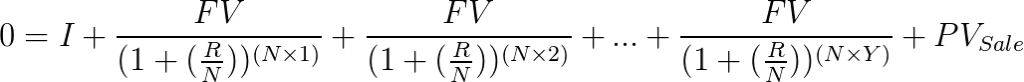Generally, solving for the rate R is an iterative process, so it’s best to use a financial calculator to come up with the answer.  Excel does this with the IRR function, and of course you can use REIkit to perform a rental property analysis.

For this investment, with static cash flows of \$10,000 each year, the IRR is 18%.

Why is that?

In this instance, even though the value of each year’s cash flow makes the investment less and less desirable each year, the reversion event of selling the property at double the price at the end of 7 years makes up for the lack in growth of the cash flows.  This deal is an appreciation play.

## Summary

When you analyze flips and wholesale deals, understanding ROI is pretty straightforward as it is easy to reason about profit vs. total costs in the short period of time that it takes you to purchase, rehab, and sell a property.

The analysis of long-term buy-and-hold rental properties often starts off similarly easy using Cash on Cash Return analysis. However, given the nature of the time value of money, CCR ends up being inadequate to describe the performance of long-term investments, especially as compared to other investments that you can potentially make.

In order to truly analyze the viability of long-term rental properties, it is important to use higher fidelity analysis models that allow for the time value of money.

You can calculate the potential profitability of your investments in comparison to your discount rate over a period of time using Net Present Value (NPV) calculations, and you can find the long-term growth rate of your investments with the Internal Rate of Return (IRR).